## Halley's Method

Also known as the Tangent Hyperbolas Method or Halley's Rational Formula. As in Halley's Irrational Formula, take the second-order Taylor Polynomial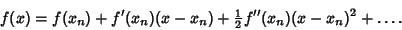(1)

A Root ofsatisfies, so(2)

Now write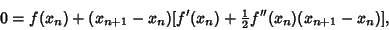(3)

giving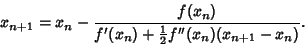(4)

Using the result from Newton's Method,(5)

gives(6)

so the iteration function is(7)

This satisfieswhereis a Root, so it is third order for simple zeros. Curiously, the third derivative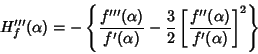(8)

is the Schwarzian Derivative. Halley's method may also be derived by applying Newton's Method to. It may also be derived by using an Osculating Curve of the form(9)

Taking derivatives,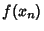(10)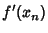(11)(12)

which has solutions(13)(14)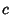(15)

so at a Root,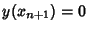and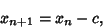(16)

which is Halley's method.

Scavo, T. R. and Thoo, J. B. On the Geometry of Halley's Method.'' Amer. Math. Monthly 102, 417-426, 1995.